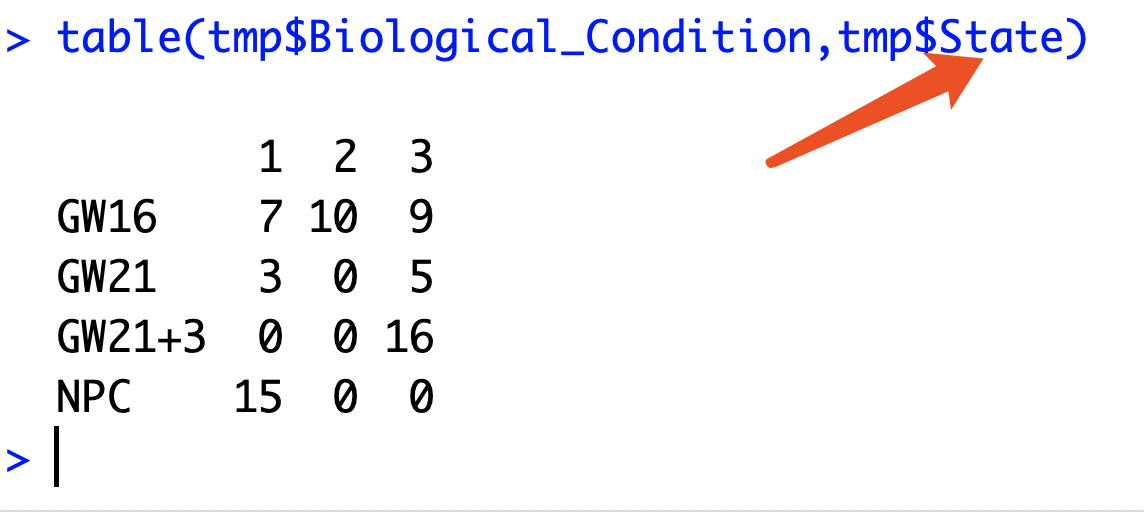# 拟时序分析后细胞类型按照不同state进行区分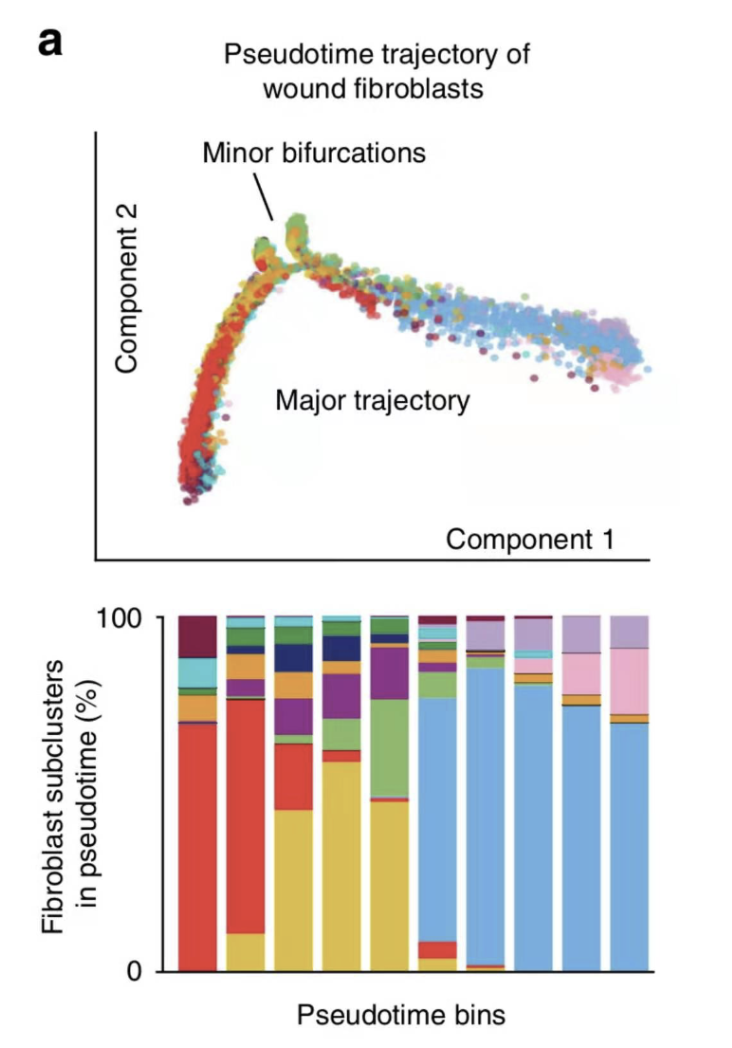### 首先我们需要再次详细理解monocle输出的细胞谱系推断图

``````load(file = 'ordering_genes_by_Biological_Condition_high.Rdata')
cds <- setOrderingFilter(cds, ordering_genes)
plot_ordering_genes(cds)
# 然后降维
cds <- reduceDimension(cds, max_components = 2,
method = 'DDRTree')
# 降维是为了更好的展示数据。
# 降维有很多种方法, 不同方法的最后展示的图都不太一样, 其中“DDRTree”是Monocle2使用的默认方法
# 接着对细胞进行排序
cds <- orderCells(cds)
## 最后两个可视化函数
plot_cell_trajectory(cds, color_by = "Biological_Condition")
``````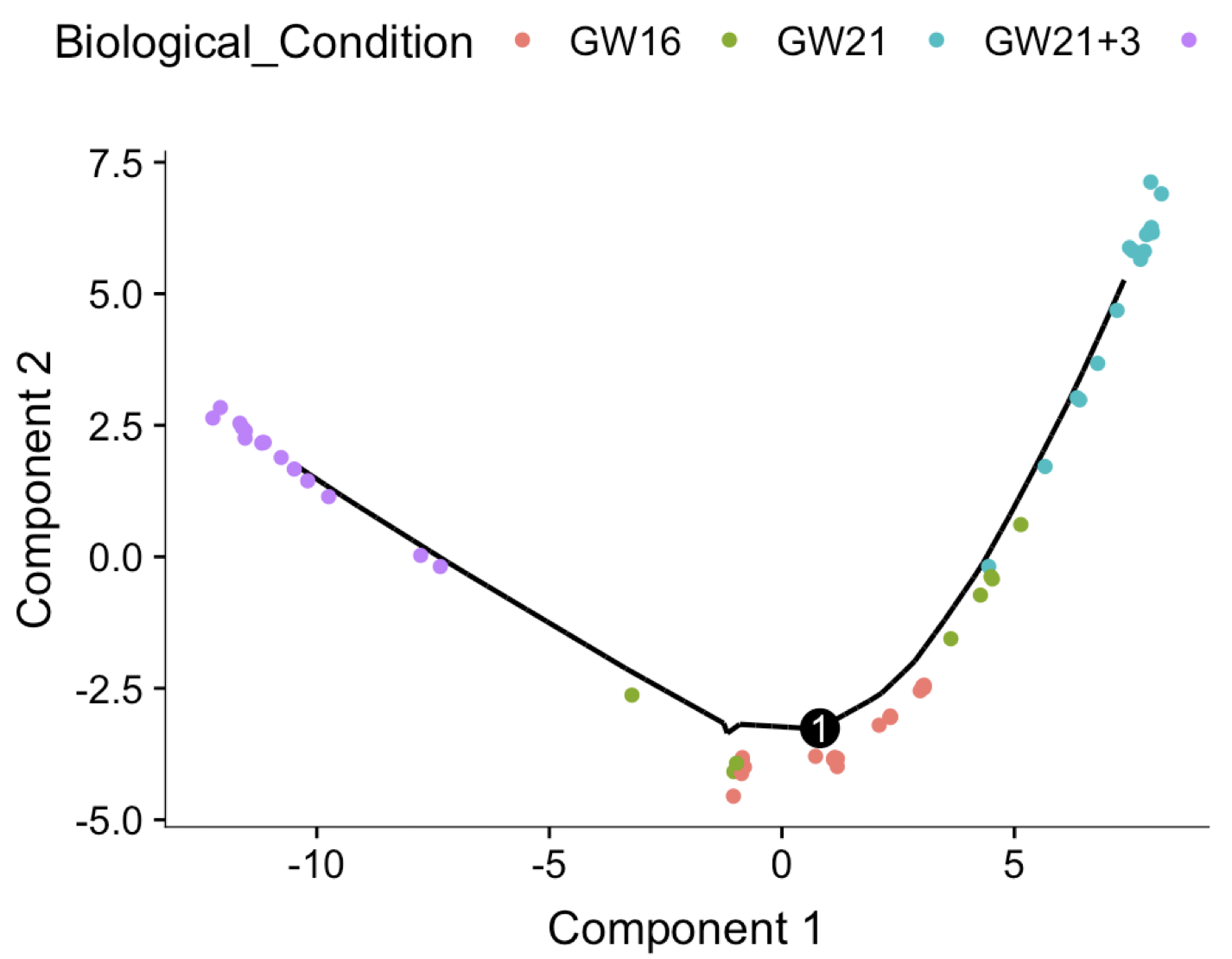### 仔细摸索 reduceDimension 函数返回值

``````Monocle provides two different algorithms for dimensionality reduction via reduceDimension. Both take a CellDataSet object and a number of dimensions allowed for the reduced space. You can also provide a model formula indicating some variables (e.g. batch ID or other technical factors) to "subtract" from the data so it doesn't contribute to the trajectory.
``````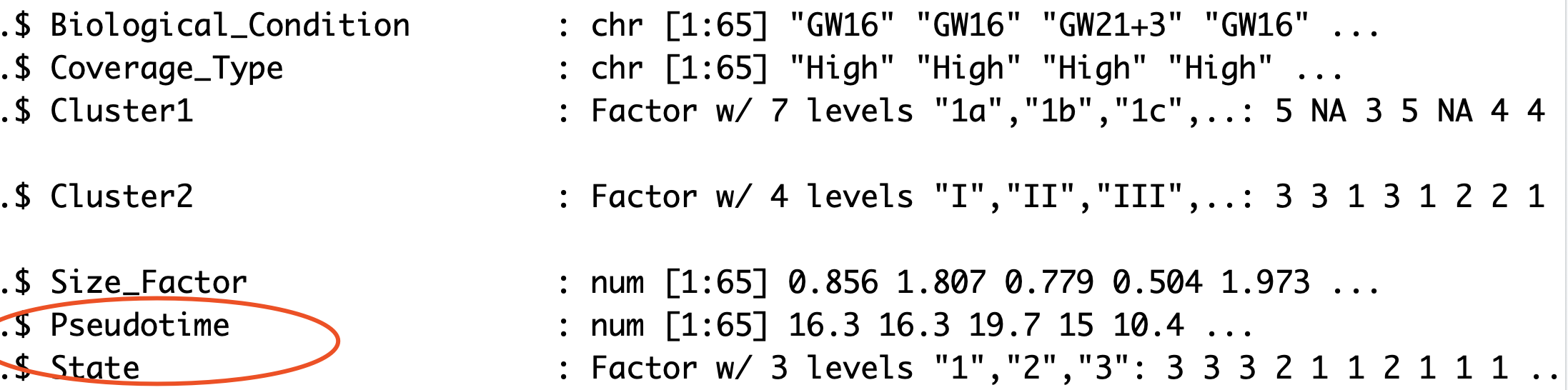``````tmp=phenoData(cds)@data
plot(tmp[,30:31])
``````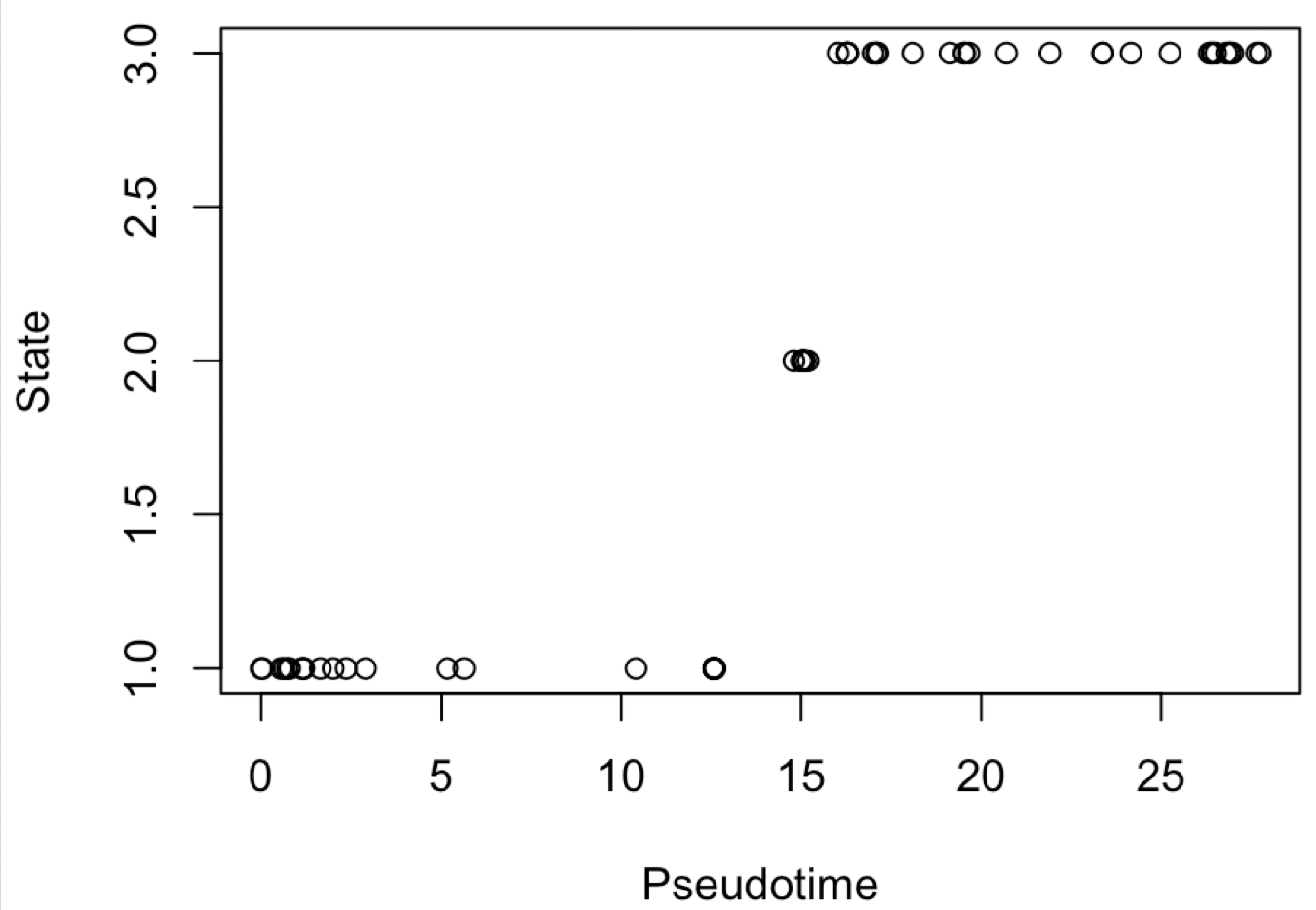### 计算Pseudotime得分的10等分里面的不同细胞类型的比例